## Grammar in Automata-

Before you go through this article, make sure that you have gone through the previous article on Types of Grammar in Automata.

On the basis of number of derivation trees, grammars are classified as-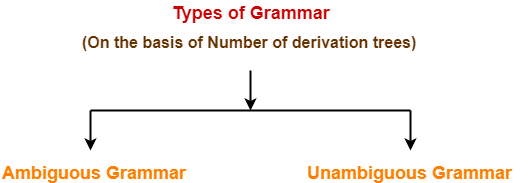1. Ambiguous Grammar
2. Unambiguous Grammar

## 1. Ambiguous Grammar-

A grammar is said to ambiguous if for any string generated by it, it produces more than one-

• Parse tree
• Or derivation tree
• Or syntax tree
• Or leftmost derivation
• Or rightmost derivation

### Example-

Consider the following grammar-

E → E + E / E x E / id

Ambiguous Grammar

This grammar is an example of ambiguous grammar.

Any of the following reasons can be stated to prove the grammar ambiguous-

### Reason-01:

Let us consider a string w generated by the grammar-

w = id + id x id

Now, let us draw the parse trees for this string w.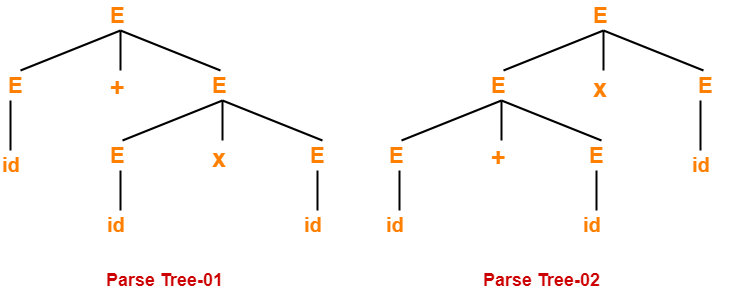Since two parse trees exist for string w, therefore the grammar is ambiguous.

### Reason-02:

Let us consider a string w generated by the grammar-

w = id + id x id

Now, let us draw the syntax trees for this string w.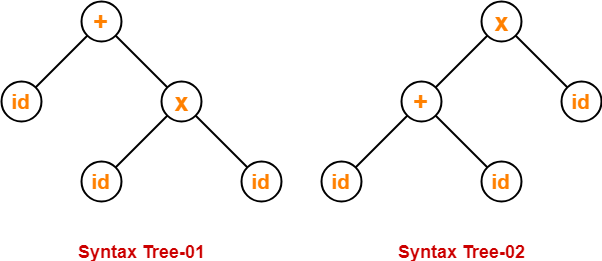Since two syntax trees exist for string w, therefore the grammar is ambiguous.

### Reason-03:

Let us consider a string w generated by the grammar-

w = id + id x id

Now, let us write the leftmost derivations for this string w.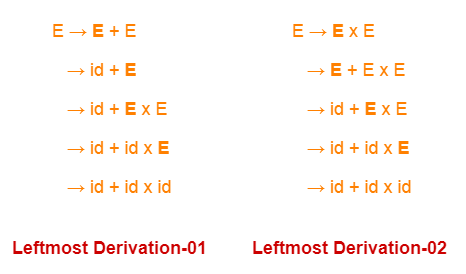Since two leftmost derivations exist for string w, therefore the grammar is ambiguous.

### Reason-04:

Let us consider a string w generated by the grammar-

w = id + id x id

Now, let us write the rightmost derivations for this string w.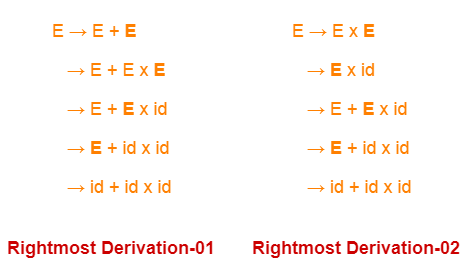Since two rightmost derivations exist for string w, therefore the grammar is ambiguous.

## 2. Unambiguous Grammar-

A grammar is said to unambiguous if for every string generated by it, it produces exactly one-

• Parse tree
• Or derivation tree
• Or syntax tree
• Or leftmost derivation
• Or rightmost derivation

### Example-

Consider the following grammar-

E → E + T / T

T → T x F / F

F → id

Unambiguous Grammar

This grammar is an example of unambiguous grammar.

To gain better understanding about Ambiguous Grammar,

Watch this Video Lecture

Next Article- Recursive Grammar

Get more notes and other study material of Theory of Automata and Computation.

Watch video lectures by visiting our YouTube channel LearnVidFun.

## Relationship Between Left Recursion, Left Factoring & Ambiguity-

 There is no relationship between Left Recursion, Left Factoring and Ambiguity of Grammar.

• All the three concepts are independent and has nothing to do with each other.
• The presence or absence of left recursion does not impact left factoring and ambiguity anyhow.
• The presence or absence of left factoring does not impact left recursion and ambiguity anyhow.
• The presence or absence of ambiguity does not impact left recursion and left factoring anyhow.

The following examples support this fact-

### Example-01: Ambiguous Grammar With Left Factoring-

Consider the following grammar-

S → aS / a / ∈

Clearly, this grammar has left factoring.

Now, let us draw parse trees for the string w = a-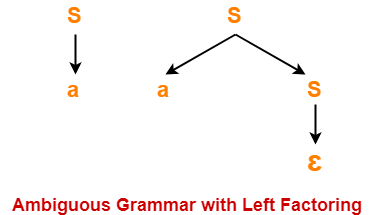Clearly,

• Two different parse trees exist for the string w = a.
• Therefore, the grammar is ambiguous.

### Example-02: Unambiguous Grammar With Left Factoring-

Consider the following grammar-

S → aA / aB

A → a

B → b

Clearly, this grammar has left factoring.

The language generated by this grammar consists of only two strings L(G) = { aa , ab}.

Now, let us draw parse trees for these strings-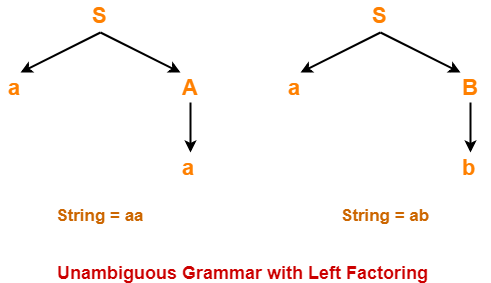Clearly,

• A unique parse tree exists for both the strings.
• Therefore, the grammar is unambiguous.

### Example-03: Ambiguous Grammar With Left Recursion-

Consider the following grammar-

S → SS / ∈

Clearly, this grammar has left recursion.

Now, let us draw parse trees for the string w = ∈-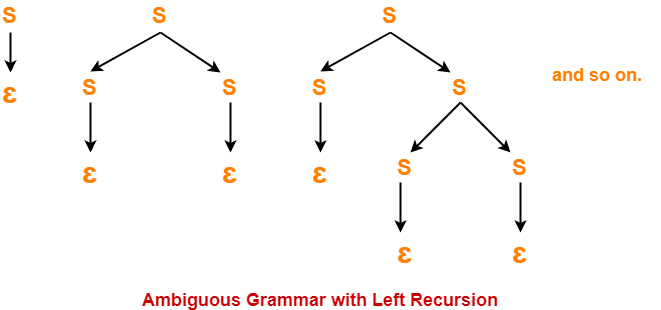Clearly,

• Infinite parse trees exist for the string w = ∈.
• Therefore, the grammar is ambiguous.

### Example-04: Unambiguous Grammar With Left Recursion-

Consider the following grammar-

S → Sa / ∈

Clearly, this grammar has left recursion.

A unique parse tree exists for all the strings that can be generated from the grammar.

Therefore, the grammar is unambiguous.

### Example-05: Ambiguous Grammar Without Left Recursion & Without Left Factoring-

Consider the following grammar-

S → aA / Ba

A → a

B → a

Clearly, this grammar has neither left recursion nor left factoring.

Now, let us draw parse trees for the string w = aa-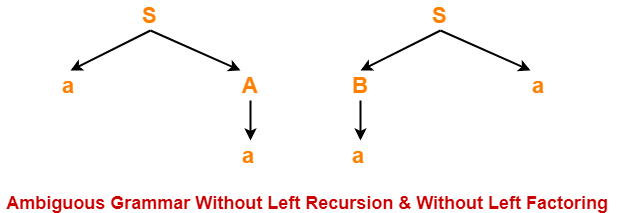Clearly,

• Two different parse trees exist for the string w = aa.
• Therefore, the grammar is ambiguous.

### Example-06: Unambiguous Grammar With Both Left Recursion & Left Factoring-

Consider the following grammar-

S → Sa / ɛ / bB / bD

B → b

D → d

Clearly, this grammar has both left recursion and left factoring.

A unique parse tree exists for all the strings that can be generated from the grammar.

Therefore, the grammar is unambiguous.

To gain better understanding about relationship between left recursion, left factoring and ambiguity-

Watch this Video LectureNext Article- Calculating First and Follow

Get more notes and other study material of Compiler Design.

Watch video lectures by visiting our YouTube channel LearnVidFun.